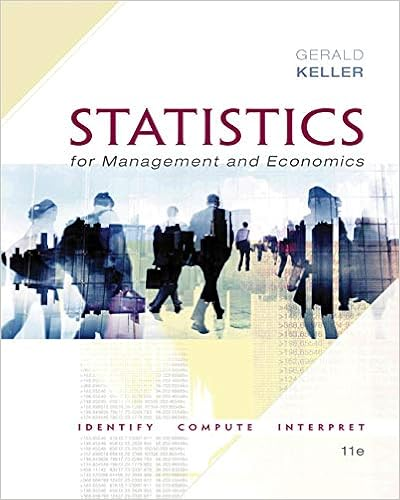# STA6126 Chapter 6 - Revised on 11:06 AM Chapter 6...

• Notes
• 12
• 100% (1) 1 out of 1 people found this document helpful

This preview shows page 1 - 4 out of 12 pages.

##### We have textbook solutions for you!
The document you are viewing contains questions related to this textbook.The document you are viewing contains questions related to this textbook.
Chapter 11 / Exercise 11.72
Statistics for Management and Economics + XLSTAT Bind-in
KellerExpert Verified
STA6126 Chapter 5, Page 1 of 12 Revised on 8/22/2011 11:06 AM Chapter 6 Statistical Inference: Significance Tests Definition:A hypothesisis a statement (sentence) with a subject (a parameter), object (a number) and a verb (one of the following: =, ≠,<, >). Hypotheses come in pairs: The Null hypothesis (Ho): is a hypothesis of no change (status quo). It represents our current knowledge about the value of the parameter.Some examples of are Ho: μ = 5, orHo: π = 0.4. The Alternative Hypothesis (Ha): is a statement of change (a claim, conjecture, guess) in the value of the parameter.Some examples are Ha: μ > 2or Ho: μ < 6 or Ha: μ ≠ 10More examples Ha: π ≠ 0.9, or Ha: π < 0.4 or Ha: π > 0.6Six Steps of Hypothesis Testing: 1.Specify the assumptions Define the random variable (population) Define the distribution of the population Define the parameter of the distribution (known and unknown) Specify data collection method and type of data Specify the sample size. 2.Specify the hypotheses: Equality is ALWAYS with Ho.Always in pairs (Ho and Ha)Ho is the hypothesis of the current knowledge (statusquo)Ha is the hypothesis of change.When one of the two hypotheses is true the other one MUST be false.3.Specify the test statistic: For testing hypotheses about the population mean,μ, the test statistic is always0(1)~/nXTtSn, where μ0is the value of μ specified by Ho.For testing hypotheses about the population proportion,π, the test statistic is always 000~(0,1)(1)pZNn
##### We have textbook solutions for you!
The document you are viewing contains questions related to this textbook.The document you are viewing contains questions related to this textbook.
Chapter 11 / Exercise 11.72
Statistics for Management and Economics + XLSTAT Bind-in
KellerExpert Verified
STA6126 Chapter 5, Page 2 of 12 4.Calculate the p-value:
)
)
)
)
)
6.Write a conclusion:Explain the decision in layman’s language with no technical jargon (except specifying the p-value or α). 6.1Tests for Population Means 1.Assumptions: a)SRS b)Quantitative variable c)Normal population d)μ = mean of the population2.Hypotheses: Ho: μ = μ0, where μ0is a number; the value of μ specified by Ho.Ha: μ ≠ μ0or Ha: μ < μ0or Ha: μ > μ03.Test Statistic: 0(1)~/nXTtSn. Remember that when the df is large, t approaches Z. 4.P-Value Depends on Ha: If Ha: μ < μ0then p-value = P(T ≤ Tcal) If Ha: μ > μ0then p-value = P(T ≥ Tcal) If Ha: μ ≠ μ0then p-value = 2 × P(T ≥ | Tcal| )
STA6126 Chapter 5, Page 3 of 12 5.Decision: General Rule: Reject Ho if p-value ≤ α.α is called the “level of significance of the test.” It is a small number, 0.01 ≤ α ≤ 0.106.Conclusion: Explain the decision in simple words.Example: In a certain area, regulations require that the chlorine level in waste water discharges be
•••